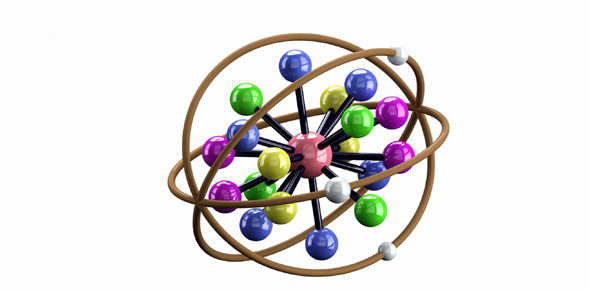# Chemical Bonds

10 QuestionsSettingsQuiz on chemical bond.

Related Topics
• 1.
Why do atoms bond?
• A.

To empty their valence shell

• B.

To become stable

• C.

To gain one more elctron

• D.

To create a new element

• 2.
What does the Octet Rule state?
• A.

Atoms lose, gain, or share electrons to obtain 9 valence electrons

• B.

Atoms always gain electrons to obtain 8 valence electrons

• C.

Atoms never change

• D.

Atoms lose, gain, or share electrons to obtain 8 valence electrons

• 3.
What do the dots on the Lewis Dot Structure represent?
• A.

The element

• B.

The type of bond

• C.

All the electrons

• D.

The valence electrons

• 4.
Which kind of bond is formed when one or more electron is shared between atoms?
• A.

Ionic bond

• B.

Covalent bond

• C.

Pi bond

• D.

Sigma bond

• 5.
What kind of bond transfers electrons?
• A.

Ionic bond

• B.

Metallic bond

• C.

Covalent bond

• D.

Polar covalent bond

• 6.
How many electrons are involved in a single covalent bond?
• A.

3

• B.

1

• C.

2

• D.

4

• 7.
What kind of bond do two hydrogen atoms form?
• A.

Covalent

• B.

Ionic

• C.

Pi

• D.

Sigma

• 8.
What is the strongest form of a covalent bond?
• A.

Single covalent bond

• B.

Pi bond

• C.

Ionic bond

• D.

Sigma bond

• 9.
In a Sigma Bond, where do the shared electrons lay?
• A.

The valence shell

• B.

Next to the nucleus of one atom

• C.

Between the nuclei

• D.

Anywhere in the shells of one atom

• 10.
Which orbital is used in a Pi Bond?
• A.

P

• B.

S

• C.

D

• D.

F